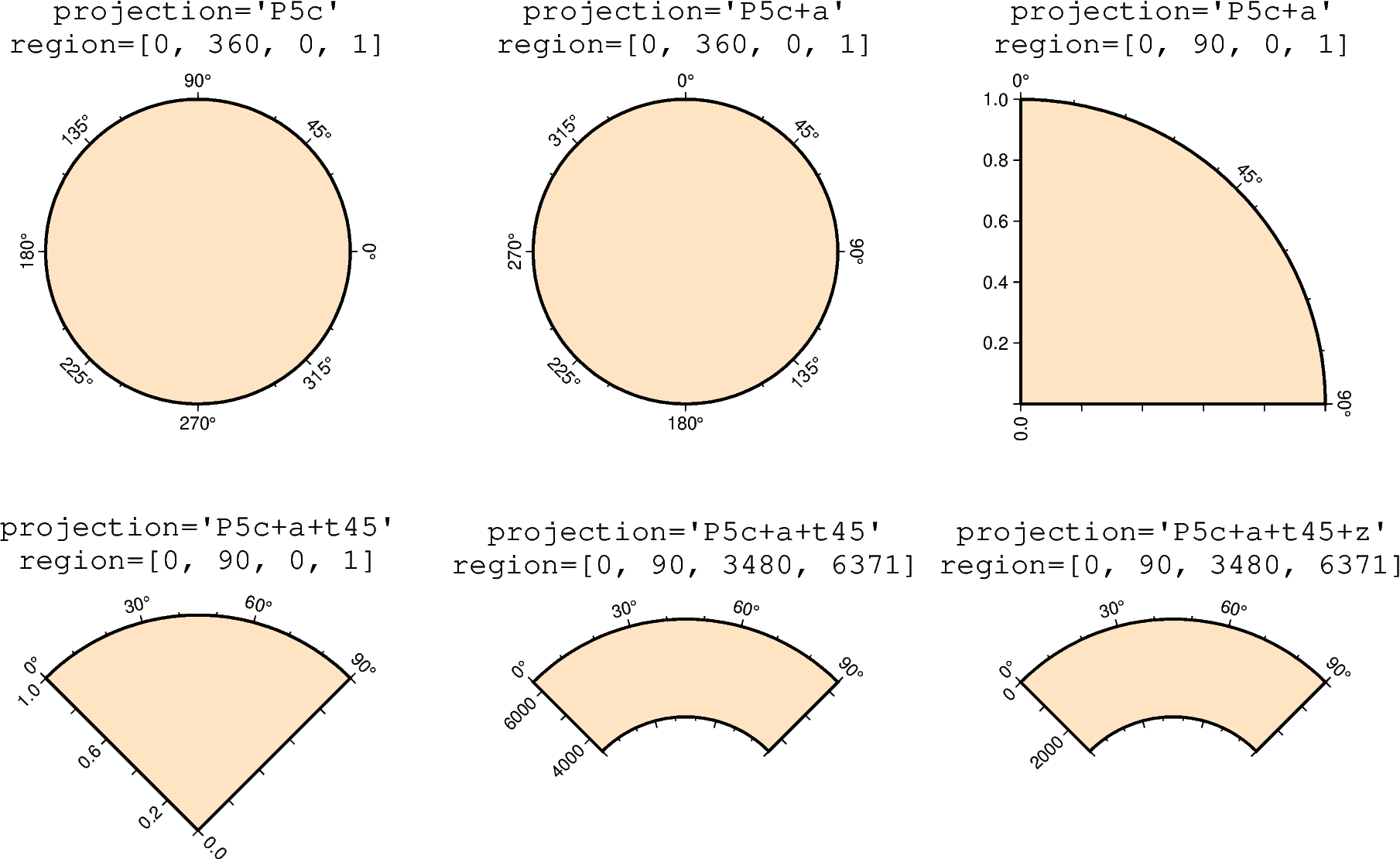# Polar

Polar projections allow plotting polar coordinate data (e.g. angle $$\theta$$ and radius r).

The full syntax for polar projections is:

Limits are set via the region parameter ([theta_min, theta_max, radius_min, radius_max]). When using Pwidth you have to give the width of the figure. The lower-case version p is similar to P but expects a scale instead of a width (pscale).

The following customizing modifiers are available:

• +a: by default, $$\theta$$ refers to the angle that is equivalent to a counterclockwise rotation with respect to the east direction (standard definition); +a indicates that the input data are rotated clockwise relative to the north direction (geographical azimuth angle).

• +roffset: represents the offset of the r-axis. This modifier allows you to offset the center of the circle from r=0.

• +torigin: sets the angle corresponding to the east direction which is equivalent to rotating the entire coordinate axis clockwise; if the +a modifier is used, setting the angle corresponding to the north direction is equivalent to rotating the entire coordinate axis counterclockwise.

• +f: reverses the radial direction.

• Append e to indicate that the r-axis is an elevation angle, and the range of the r-axis should be between 0° and 90°.

• Appending p sets the current Earth radius (determined by PROJ_ELLIPSOID) to the maximum value of the r-axis when the r-axis is reversed.

• Append radius to set the maximum value of the r-axis.

• +z: indicates that the r-axis is marked as depth instead of radius (e.g. r = radius - z).import pygmt

fig = pygmt.Figure()

pygmt.config(FONT_TITLE="14p,Courier,black", FORMAT_GEO_MAP="+D")

# ============
# top left
fig.basemap(
# set map limits to theta_min = 0, theta_max = 360, radius_min = 0,
region=[0, 360, 0, 1],
# set map width to 5 cm
projection="P5c",
# set the frame, color, and title
# @^ allows for a line break within the title
frame=["xa45f", "+gbisque+tprojection='P5c' @^ region=[0, 360, 0, 1]"],
)

fig.shift_origin(xshift="8c")

# ============
# top middle
fig.basemap(
# set map limits to theta_min = 0, theta_max = 360, radius_min = 0,
region=[0, 360, 0, 1],
# set map width to 5 cm and interpret input data as geographic azimuth
projection="P5c+a",
# set the frame, color, and title
# @^ allows for a line break within the title
frame=["xa45f", "+gbisque+tprojection='P5c+a' @^ region=[0, 360, 0, 1]"],
)

fig.shift_origin(xshift="8c")

# ============
# top right
fig.basemap(
# set map limits to theta_min = 0, theta_max = 90, radius_min = 0,
region=[0, 90, 0, 1],
# set map width to 5 cm and interpret input data as geographic azimuth
projection="P5c+a",
# set the frame, color, and title
# @^ allows for a line break within the title
frame=["xa45f", "ya0.2", "WNe+gbisque+tprojection='P5c+a' @^ region=[0, 90, 0, 1]"],
)

fig.shift_origin(xshift="-16c", yshift="-7c")

# ============
# bottom left
fig.basemap(
# set map limits to theta_min = 0, theta_max = 90, radius_min = 0,
region=[0, 90, 0, 1],
# set map width to 5 cm and interpret input data as geographic azimuth
# instead of standard angle, rotate coordinate system counterclockwise by
# 45 degrees
projection="P5c+a+t45",
# set the frame, color, and title
# @^ allows for a line break within the title
frame=[
"xa30f",
"ya0.2",
"WNe+gbisque+tprojection='P5c+a+t45' @^ region=[0, 90, 0, 1]",
],
)

fig.shift_origin(xshift="8c", yshift="1.3c")

# ============
# bottom middle
fig.basemap(
# set map limits to theta_min = 0, theta_max = 90, radius_min = 3480,
region=[0, 90, 3480, 6371],
# set map width to 5 cm and interpret input data as geographic azimuth
# instead of standard angle, rotate coordinate system counterclockwise by
# 45 degrees
projection="P5c+a+t45",
# set the frame, color, and title
# @^ allows for a line break within the title
frame=[
"xa30f",
"ya",
"WNse+gbisque+tprojection='P5c+a+t45' @^ region=[0, 90, 3480, 6371]",
],
)

fig.shift_origin(xshift="8c")

# ============
# bottom right
fig.basemap(
# set map limits to theta_min = 0, theta_max = 90, radius_min = 3480,
region=[0, 90, 3480, 6371],
# set map width to 5 cm and interpret input data as geographic azimuth
# instead of standard angle, rotate coordinate system counterclockwise by
# 45 degrees, r-axis is marked as depth
projection="P5c+a+t45+z",
# set the frame, color, and title
# @^ allows for a line break within the title
frame=[
"xa30f",
"ya",
"WNse+gbisque+tprojection='P5c+a+t45+\\z' @^ region=[0, 90, 3480, 6371]",
],
)

fig.show()


Total running time of the script: ( 0 minutes 1.651 seconds)

Gallery generated by Sphinx-Gallery